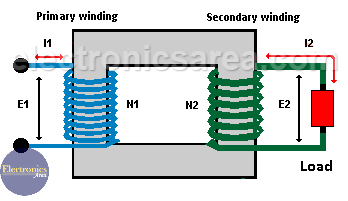# Transformer Turn Ratio (TTR)

## Transformer turn ratio K using winding number of turns

The transformer transformation ratio or transformer turns ratio (K) is the quotient value obtained by dividing the number of turns of the primary winding (N1) and the number of turns of the secondary winding (N2). Then K = N1/N2.

## Transformer turn ratio K using electromotive forces

In a transformer with no load connected to the secondary winding, the induced electromotive forces (E1 and E2) have a direct relationship between them. So we can also obtain the transformer transformation ratio, using E1 and E2. Then: K = E1/E2.

## Transformer turn ratio K using winding currents

Another way to find the transformation ratio of a transformer is using the nominal currents of the primary winding and the secondary winding (I1 and I2) based on the inverse relationship to the electromotive force. K = I2 / I1.

On the diagram above parameters N1, N2, E1, E2, I1 and I2 are shown. From the above, we can summarize that the transformation ratio is obtained by: K = N1/N2 = E1/E2 = I2/I1.The latter equation allows us to obtain the transformation ratio K by different means. It also allows us to obtain the values of voltage,current and number of turns in the primary and secondary winding (N1 and N2), if we know the value of K and one or more other parameters.

## The Ideal Transformer and the Transformer transformation ratio

In a real transformer, there are voltage drops due to the resistance in the winding and losses in the magnetic flux of the transformer core. So:

V1 = E1 + voltage drop
E2 = V2 + voltage drop

where:

V = voltage in the respective coil
E = emf in the respective coil

In the ideal case, these losses can be neglected.

Hence, K = E2/E1 = V2/V1 = I2/I1 = N2/N1.

•
•
•
•
•
•
•
•
•
•
•
•
•
•
•
•
•
•
Tagged with: